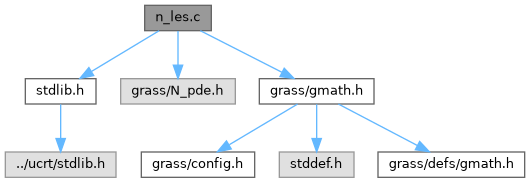GRASS GIS 8 Programmer's Manual  8.2.2dev(2023)-e6660eef60
n_les.c File Reference
`#include <stdlib.h>`
`#include <grass/N_pde.h>`
`#include <grass/gmath.h>`
Include dependency graph for n_les.c:Go to the source code of this file.

## Functions

N_lesN_alloc_nquad_les (int cols, int rows, int type)
Allocate memory for a (not) quadratic linear equation system which includes the Matrix A, vector x and vector b. More...

N_lesN_alloc_nquad_les_Ax (int cols, int rows, int type)
Allocate memory for a (not) quadratic linear equation system which includes the Matrix A and vector x. More...

N_lesN_alloc_nquad_les_A (int cols, int rows, int type)
Allocate memory for a (not) quadratic linear equation system which includes the Matrix A. More...

N_lesN_alloc_nquad_les_Ax_b (int cols, int rows, int type)
Allocate memory for a (not) quadratic linear equation system which includes the Matrix A, vector x and vector b. More...

N_lesN_alloc_les (int rows, int type)
Allocate memory for a quadratic linear equation system which includes the Matrix A, vector x and vector b. More...

N_lesN_alloc_les_Ax (int rows, int type)
Allocate memory for a quadratic linear equation system which includes the Matrix A and vector x. More...

N_lesN_alloc_les_A (int rows, int type)
Allocate memory for a quadratic linear equation system which includes the Matrix A. More...

N_lesN_alloc_les_Ax_b (int rows, int type)
Allocate memory for a quadratic linear equation system which includes the Matrix A, vector x and vector b. More...

N_lesN_alloc_les_param (int cols, int rows, int type, int parts)
Allocate memory for a quadratic or not quadratic linear equation system. More...

void N_print_les (N_les *les)
prints the linear equation system to stdout More...

void N_free_les (N_les *les)
Release the memory of the linear equation system. More...

## ◆ N_alloc_les()

 N_les* N_alloc_les ( int rows, int type )

Allocate memory for a quadratic linear equation system which includes the Matrix A, vector x and vector b.

This function calls N_alloc_les_param

Parameters
 rows int type int
Returns
N_les *

Definition at line 100 of file n_les.c.

## ◆ N_alloc_les_A()

 N_les* N_alloc_les_A ( int rows, int type )

Allocate memory for a quadratic linear equation system which includes the Matrix A.

This function calls N_alloc_les_param

Parameters
 rows int type int
Returns
N_les *

Definition at line 130 of file n_les.c.

## ◆ N_alloc_les_Ax()

 N_les* N_alloc_les_Ax ( int rows, int type )

Allocate memory for a quadratic linear equation system which includes the Matrix A and vector x.

This function calls N_alloc_les_param

Parameters
 rows int type int
Returns
N_les *

Definition at line 115 of file n_les.c.

## ◆ N_alloc_les_Ax_b()

 N_les* N_alloc_les_Ax_b ( int rows, int type )

Allocate memory for a quadratic linear equation system which includes the Matrix A, vector x and vector b.

This function calls N_alloc_les_param

Parameters
 rows int type int
Returns
N_les *

Definition at line 145 of file n_les.c.

## ◆ N_alloc_les_param()

 N_les* N_alloc_les_param ( int cols, int rows, int type, int parts )

The type of the linear equation system must be N_NORMAL_LES for a regular quadratic matrix or N_SPARSE_LES for a sparse matrix

In case of N_NORMAL_LES

A quadratic matrix of size rows*rows*sizeof(double) will allocated

In case of N_SPARSE_LES

a vector of size row will be allocated, ready to hold additional allocated sparse vectors. each sparse vector may have a different size.

Parameter parts defines which parts of the les should be allocated. The number of columns and rows defines if the matrix is quadratic.

Parameters
 cols int rows int type int parts int – 2 = A, x and b; 1 = A and x; 0 = A allocated
Returns
N_les *

Definition at line 178 of file n_les.c.

 N_les* N_alloc_nquad_les ( int cols, int rows, int type )

Allocate memory for a (not) quadratic linear equation system which includes the Matrix A, vector x and vector b.

This function calls N_alloc_les_param

Parameters
 cols int rows int type int
Returns
N_les *

Definition at line 35 of file n_les.c.

 N_les* N_alloc_nquad_les_A ( int cols, int rows, int type )

Allocate memory for a (not) quadratic linear equation system which includes the Matrix A.

This function calls N_alloc_les_param

Parameters
 cols int rows int type int
Returns
N_les *

Definition at line 67 of file n_les.c.

 N_les* N_alloc_nquad_les_Ax ( int cols, int rows, int type )

Allocate memory for a (not) quadratic linear equation system which includes the Matrix A and vector x.

This function calls N_alloc_les_param

Parameters
 cols int rows int type int
Returns
N_les *

Definition at line 51 of file n_les.c.

 N_les* N_alloc_nquad_les_Ax_b ( int cols, int rows, int type )

Allocate memory for a (not) quadratic linear equation system which includes the Matrix A, vector x and vector b.

This function calls N_alloc_les_param

Parameters
 cols int rows int type int
Returns
N_les *

Definition at line 83 of file n_les.c.

## ◆ N_free_les()

 void N_free_les ( N_les * les )

Release the memory of the linear equation system.

Parameters
 les N_les *
Returns
void

Definition at line 304 of file n_les.c.

## ◆ N_print_les()

 void N_print_les ( N_les * les )

prints the linear equation system to stdout

Format: A*x = b

Example

```2 1 1 1 * 2 = 0.1
1 2 0 0 * 3 = 0.2
1 0 2 0 * 3 = 0.2
1 0 0 2 * 2 = 0.1```
Parameters
 les N_les *
Returns
void

Definition at line 252 of file n_les.c.